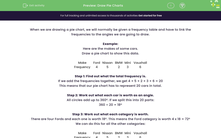# Draw Pie Charts

In this worksheet, students will practise drawing pie charts.Key stage:  KS 4

Year:  GCSE

GCSE Subjects:   Maths

GCSE Boards:   AQA, Eduqas, Pearson Edexcel, OCR,

Curriculum topic:   Statistics

Curriculum subtopic:   Statistics Interpreting and Representing Data

Difficulty level:#### Worksheet Overview

When we are drawing a pie chart, we will normally be given a frequency table and have to link the frequencies to the angles we are going to draw.

Example:

Here are the makes of some cars.

Draw a pie chart to show this data.

 Make Ford Nissan BMW Mini Vauxhall Frequency 4 5 2 3 6

Step 1: Find out what the total frequency is.

If we add the frequencies together, we get 4 + 5 + 2 + 3 + 6 = 20

This means that our pie chart has to represent 20 cars in total.

Step 2: Work out what each car is worth as an angle.

All circles add up to 360°. If we split this into 20 parts:

360 ÷ 20 = 18°

Step 3: Work out what each category is worth.

There are four Fords and each one is worth 18°. This means the Ford category is worth 4 x 18 = 72°

We can do this for all the other categories:

 Make Ford Nissan BMW Mini Vauxhall Frequency 4 5 2 3 6 Angle 72 90 36 54 108

We can now check we have done this correctly by adding up all the angles. We should always get 360.

Step 4: Draw the angles onto a pie chart and label the sectors.

You can either give a key, or write the names in the sectors. It doesn't matter which you do, you'll still get the same marks either way.Let's move on to some questions now.

### What is EdPlace?

We're your National Curriculum aligned online education content provider helping each child succeed in English, maths and science from year 1 to GCSE. With an EdPlace account you’ll be able to track and measure progress, helping each child achieve their best. We build confidence and attainment by personalising each child’s learning at a level that suits them.

Get started